# Convex function (of a real variable)

(diff) ← Older revision | Latest revision (diff) | Newer revision → (diff)

A function, defined on some interval, satisfying the condition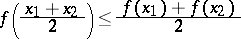(1)

for every two points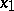and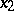from this interval. The geometrical meaning of this condition is that the midpoint of any chord of the graph of the functionis located either above the graph or on it. If the inequality (1) is strict for alland, thenis called strictly convex. Examples of convex functions include,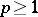,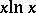for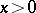, and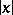for all. If the sign of inequality (1) is reversed, the function is called concave. All measurable convex functions on open intervals are continuous. There exist convex functions which are not continuous, but they are very irregular: If a functionis convex on the intervaland is bounded from above on some interval lying inside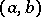, it is continuous on. Thus, a discontinuous convex function is unbounded on any interior interval and is not measurable.

If a functionis continuous on an interval, and if each chord of its graph contains at least one point other than the end points of the chord and lying above the graph or on it,is convex. It follows from condition (1) that for a continuous function the centre of gravity of any finite number of material points lying on the graph of the function lies either above the graph or on it: For any numbers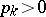,(whereis arbitrary), the Jensen inequality(2)

is valid.

If, for some function, inequality (2) is true for any two pointsandin some interval and any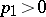and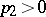, the functionis continuous and, of course, convex on this interval. Any chord of the graph of a continuous convex function coincides with the corresponding part of the graph or lies entirely above the graph except for its end points. This means that if a continuous convex function is not linear on any interval, strict inequality is realized in (1) and (2) for any pairwise different values of the argument, i.e.is a strictly convex function.

A continuous function is convex if and only if the set of points of the plane located above its graph, i.e. its supergraph, is a convex set. For a continuous function, defined on an interval, to be convex, it is necessary and sufficient that for each point on the graph there be at least one straight line (known as a supporting line) situated under the graph (on the interval) or partly on the graph that passes through the point, i.e. for any point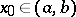there exists asuch that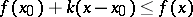(3)

for all.

A continuous function which is convex on an open interval has no strict local maximum. If a functionis continuous and convex on an interval, it has, at each one of its points, a finite left,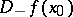, and right,, derivative; moreover,and, in addition, if the number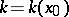satisfies condition (3), the inequalities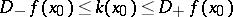hold. The functions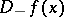anddo not decrease, and at all points except, possibly, a countable number of them,, so thatis differentiable at these points. On each closed interval located insidethe functionsatisfies a Lipschitz condition and is thus absolutely continuous. This makes it possible to establish the following convexity criterion: A continuous function is convex if and only if it is the indefinite integral of a non-decreasing function.

If a functionis differentiable on an interval, it is (strictly) convex on this interval if and only if its derivative does not decrease (is increasing). At a point of the graph of a continuous convex function at which the function is differentiable there exists a unique supporting line — the tangent at this point. On the other hand, if, at any point of the graph of a function which is differentiable on an interval, the tangent to the graph at that point lies under the graph in some neighbourhood of that point (except at the tangency point itself), the function is strictly convex; if it lies under the graph or partly on it, it is just a convex function.

If the functionis twice-differentiable on the interval, it is convex on this interval if and only if its second derivative is non-negative on this interval (this theorem is valid for the second symmetric derivative, as well as for the ordinary second derivative). If the function has a positive second derivative at each point of some interval, it is strictly convex on that interval.

If the functions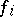are convex on an intervaland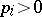,, then the function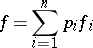is also convex on this interval; also, if even one of the functionsis strictly convex,is strictly convex as well.

There exist various generalizations of the concept of convexity to functions of several variables. For instance, let a function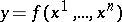be defined on a convex setof the-dimensional affine space. The functionis called convex if inequality (1) is valid for all pointsand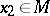, wheredenotes the sum of the-dimensional vectorsand. The properties of a convex function of one variable are correspondingly generalized to functions of several variables; for example, inequality (2) is satisfied only for continuous convex functions. A continuous function is convex if and only if the set of points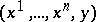of the spacelying above its graph is convex.

A continuous functiondefined on a convex domainis convex if and only if for each point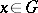there exists a linear function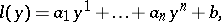such that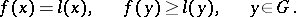(4)

The hyperplane defined by the equation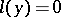is called a supporting hyperplane.

If a functionis continuously differentiable in, condition (4) is equivalent to the conditionIfis twice-differentiable, condition (4) is equivalent to the condition that the second differential of the function, i.e. the quadratic form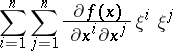is non-negative for all.

Another important generalization of the concept of a convex function for functions of several variables is the concept of a subharmonic function. The concept of a convex function can be extended in a natural manner to include functions defined on corresponding subsets of infinite-dimensional linear spaces; cf. Convex functional.

How to Cite This Entry:
Convex function (of a real variable). Encyclopedia of Mathematics. URL: http://encyclopediaofmath.org/index.php?title=Convex_function_(of_a_real_variable)&oldid=15815
This article was adapted from an original article by L.D. Kudryavtsev (originator), which appeared in Encyclopedia of Mathematics - ISBN 1402006098. See original article